### Home > CC2 > Chapter 2 > Lesson 2.2.2 > Problem2-51

2-51.

Write an expression to represent each of the following sketches. Then give the value of each sketch. Homework Help ✎

1.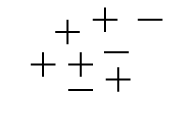Look for pairs of positive and negative integers that will add to zero.

This will be easier if you line up the symbols, as below.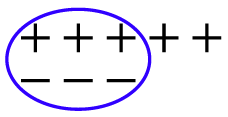Write an expression representing the integers that added to zero, plus the remaining integers.

$0+0+0+1+1$

One solution to this problem is $0+0+0+1+1=2$.
You can also write this as $5+(-3)=2$.

1.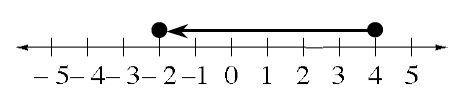Where does the arrow begin? Where does it end? How long is the arrow?

$4-6=-2$ or $4+(-6)=-2$

1.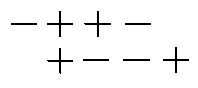How many $+$'s are there? How many $-$'s? How many zeros? When you eliminate the zeros, what is left over?

1.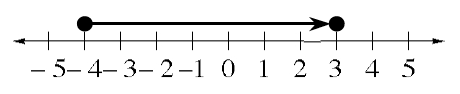See the help for part (b).